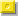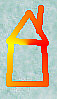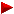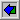HOME plateWON | World!OfNumbersDetails ofPalindromic Quasi_Over_SquaresPalindromic Quasi_Over_Squares

Legend

F_qs Decomposition of the quasi_over_square in its prime factors. Date Date of discovery by the author.Hypertext links to related topics in number theory Comm Comments about the palindromic Quasi_Over_Square number.

FactorizationsF_qs  30.103 =
PRIME

Comm 30103 is the only known multi-digit palindromic prime of the form sigma(n)/d(n), when n is composite. ( McCranie and Honaker ) sigma(n) = the sum of all the factors of n, including 1 and n itself. d(n) = the number of factors of n, including 1 and n itself. 30103 is also nice because it comes up for n = 149645 (an odd number) and once for n = 179574 (an even number). n sigma(n) d(n) sigma(n)/d(n)=palprime 149645 180618 6 30103 179574 361236 12 30103 30103 is still the only "known" palprime with the property.

[TOP OF PAGE]

Patrick De Geest - Belgium- Short Bio - Some Pictures